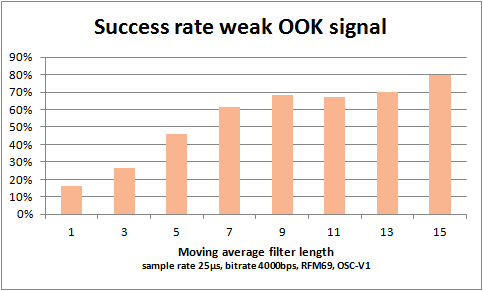# Evaluation noise filtering weak OOK signals – RFM69

As explained in a previous post , noise can spoil the reception of OOK signals from remote sensors. One solution to deal with the noise filtering of spikes in the ON/OFF binary signals is to apply a moving average filter of the last n received samples. When n is odd, the “average” is one if more then half the bits in the filter buffer is one, and otherwise zero. With a filter length of n=3 a single outlier sample will not disturb the intended signal. With a filter length of 5, spikes with a duration of two samples can be handled. Clean signals are as snappy as the where, and timings can be determined to the accuracy of the sample rate.

To find the optimal length of the moving average filter, a setup was created with a remote sensor giving a signal that was not received all the time. For each filter length, 100 transmissions where listened to. The graph below shows the success rate as function of the filter length.The binary ON/OFF signal was sampled on the DATA/DIO2 pin of the RFM69 with sample rate 25µs. The bitrate was set to 4000bps. With this setting, the internal sampling rate of the RFM69 is 10µs. This “guarantees” a noisy signal for test purposes. The filter length n=1 is actually unfiltered data at 25µs/sample offered to the decoders. The longest filter length n=15 takes an averaging window of 375µs. This is resiliant to noisy spikes (either ON or OFF) of 7 times the sample rate, thus 175µs. The minimum pulse duration of the received signal, the Oregon Scientific V1 sensor, is about 1100µs, and obviously can stand the long filter periods.

For OOK signals that vary quicker, possibly a shorter filter length should be taken. The shortest pulse durations of OOK sensors and remote controls is around 300µs.

The design of the filter is simply an unbsigned integer, in which the most recent reading (1 or 0) is shifted in from the right. The filter length is enforced by only looking at the least significant n bits. Deciding on the filtered value is a matter of counting the one-bits in the filter.

```//moving average buffer, max length = 31!
static uint32_t filter= 0;
static uint8_t avg_len = 7;
static uint32_t sig_mask = (1 << avg_len) - 1;

while (....) {
filter = (filter << 1) | (data_in & 0x01);

//efficient bit count, from literature
uint8_t c = 0;
uint32_t v = filter & sig_mask;
for (c = 0; v; c++)
{
v &= v - 1; // clear the least significant bit set
}
uint8_t data_out = (c > (avg_len >> 1)); //bit count moving average

//decode OOK signal
}
}
```